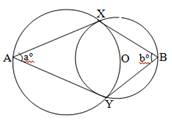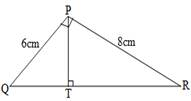Home Technical Mathematics Languages Science Social Science Art Literature Arabic Islamic Studies C.R.K HistoryMusicVisual Art Clothing/Textile Home Management Shorthand
 General Mathematics Paper 2, Nov/Dec. 2009
 Questions: 1 2 3 4 5 6 7 8 9 10 11 12 13 Main
Weakness/Remedies
Strength
Question 5

(a)In the diagram, the two circles intersect at X and Y.  The centre of the smaller circle is on the circumference of the bigger circle. A and B are any two points on the major arcs, one on each circle.  Find an equation connecting a and b.

(b)In the diagram <QPR = <PTR = 90°, /PR/ = 8cm and /QP/ = 6cm.  Find /TR/.

_____________________________________________________________________________________________________
Observation

The report stated that majority of the candidates could not answer part (a) of this question correctly which showed a poor knowledge of circle theorems.  They were expected to join X and Y to O.  This made <XOY and <XAY opposite angles of a cyclic quadrilateral and are supplementary i.e. a° + <XOY = 180°.  Also, <XOY = 2b° i.e. angle at the centre of a circle is twice angle at the circumference.  This implied that 2b° = 180 - a° or a° + 2b°  = 180°.
It was reported that candidates performed better in part (b) than in part (a).  A good number of them were able to find the tangent of the angle at R and hence the angle at R as 36.87°.  Thus, they were able to obtain /TR/ = 8 cos R i.e. 8 cos 36.87° = 6.4cm.